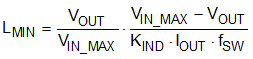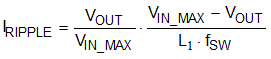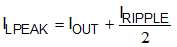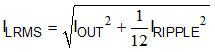SLUSDX1A September   2020  – August 2021

PRODUCTION DATA

1. Features
2. Applications
3. Description
4. Revision History
5. Pin Configuration and Functions
6. Specifications
7. Detailed Description
1. 7.1 Overview
2. 7.2 Functional Block Diagram
3. 7.3 Feature Description
4. 7.4 Device Functional Modes
8. Application and Implementation
1. 8.1 Application Information
2. 8.2 Typical Application
9. Power Supply Recommendations
10. 10Layout
11. 11Device and Documentation Support
12. 12Mechanical, Packaging, and Orderable Information

• DRL|8

#### 8.2.2.3 Output Inductor Selection

To calculate the minimum value of the output inductor, use Equation 12. KIND is a coefficient that represents the amount of inductor ripple current relative to the maximum output current. The inductor ripple current is filtered by the output capacitor. Therefore, choosing high inductor ripple currents impact the selection of the output capacitor because the output capacitor must have a ripple current rating equal to or greater than the inductor ripple current. In general, the inductor ripple value is at the discretion of the designer. For this part, TI recommends the range of KIND from 25% to 55%.

Equation 12.where

• IOUT = 3 A

For this design example, use KIND = 50%. The inductor value is calculated to be 2.99 μH. For this design, a nearest standard value was chosen: 3.3 μH. For the output filter inductor, it is important that the RMS current and saturation current ratings not be exceeded. The inductor peak-to-peak ripple current, peak current, and RMS current are calculated using Equation 13, Equation 14, and Equation 15.

Equation 13.Equation 14.Equation 15.For this design example, the calculated peak current is 3.68 A and the calculated RMS current is 3.02 A. The chosen inductor is a Wurth Elektronik 74437349033 3.3-μH. It has a saturation current rating of 12 A and a RMS current rating of 6 A.

The current flowing through the inductor is the inductor ripple current plus the output current. During power up, faults, or transient load conditions, the inductor current can increase above the calculated peak inductor current level calculated above. In transient conditions, the inductor current can increase up to the switch current limit of the device. For this reason, the most conservative approach is to specify an inductor with a saturation current rating equal to or greater than the switch current limit rather than the peak inductor current.